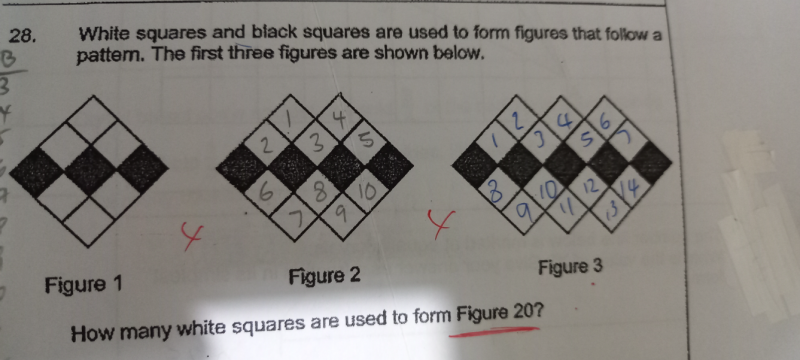# QuestionThere are many ways to approach this problem and I like to simplify the problem.
If we observe the expanding figure we would notice that the top white squares equal the bottom white squares so let’s just look at the top ones, figure out the pattern and scale later.

The number of white squares at the top follow a pattern:

fig 1. 3

fig 2. 3 + 2

fig 3. 3 + 4

Coding this pattern we have:

fig 1. 3 + (2 x 0)

fig 2. 3 + (2 x 1)

fig 3. 3 + (2 x 2)

The changing number is always 1 less than the fig’s number.

therefore at fig 20, we should expect:

fig 20. 3 + (2 x 19) = 41

Remembering to include the bottom squares,

2 x 41 = 82

hope this method is easy to relate to.

0 Replies 1 Like

Alternative Solution:

Fig                  White

1                           6

2                         10

3                         14

:                          :

n                         ?

This is a series pattern of 6,10,14,………

The standard nth term generator = first term + ( previous term x difference)

The difference between each term is 4.

The first term is 6

nth term = 6 + (n-1) x 4 = 6 + 4n- 4 = 4n + 2

Figure 20 = 4(20) + 2 = 82.

0 Replies 1 Like

The number of black square is equal to figure number +2 and therefore at figure 20, the black square number is 20+2=22.

The number of white square above and below the black square are :

the number of black squares – 1= 22-1 = 21

so 21 X 2= 42

The number of white squares on the top and the bottom row are :

the number of black squares – 2 = 22-2 =20

so 20 X 2 =40

Total whites squares at figure 20 = 40 + 42 = 82

Correct me if I made any mistake on the above.  Thank you.

0 Replies 1 Like